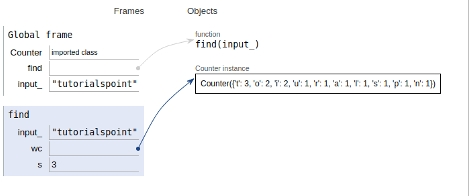# Python program to find the most occurring character and its count

In this article, we will learn about the solution and approach to solve the given problem statement.

## Problem statement

Given an input string we need to find the most occurring character and its count.

## Approach

• Create a dictionary using Counter method having strings as keys and their frequencies as values.

• Find the maximum occurrence of a character i.e. value and get the index of it.

Now let’s see the implementation below −

## Example

from collections import Counter
def find(input_):
# dictionary
wc = Counter(input_)
# Finding maximum occurrence
s = max(wc.values())
i = wc.values().index(s)
print (wc.items()[i])
# Driver program
if __name__ == "__main__":
input_ = 'Tutorialspoint'
find(input_)

## Output

(‘t’,3)

All the variables and functions are declared in the global scope as shown below −## Conclusion

In this article, we learnt about the approach to find the most occurring character and its count.

Updated on: 26-Sep-2019

340 Views